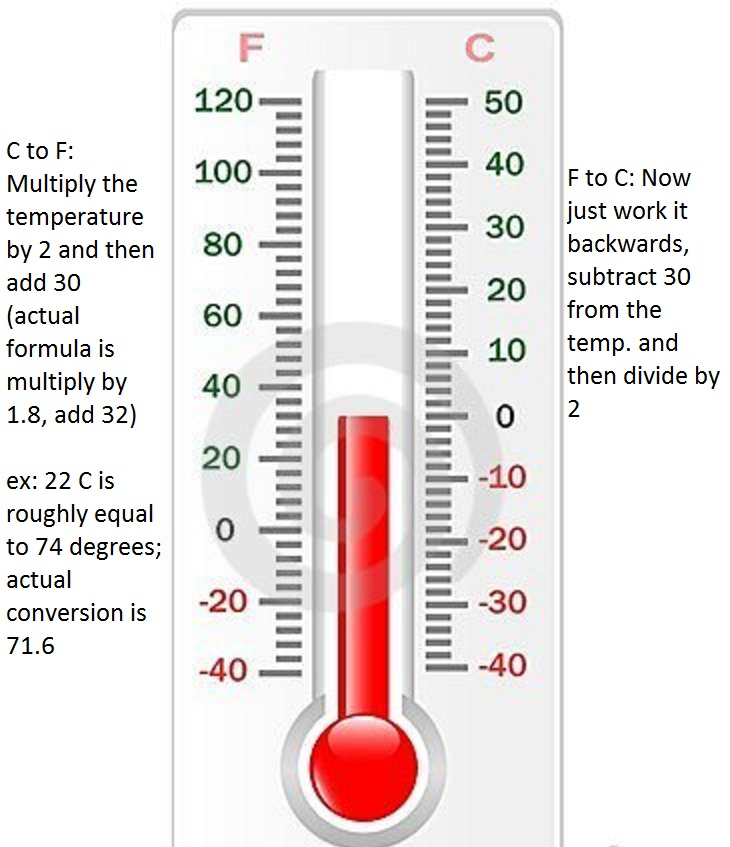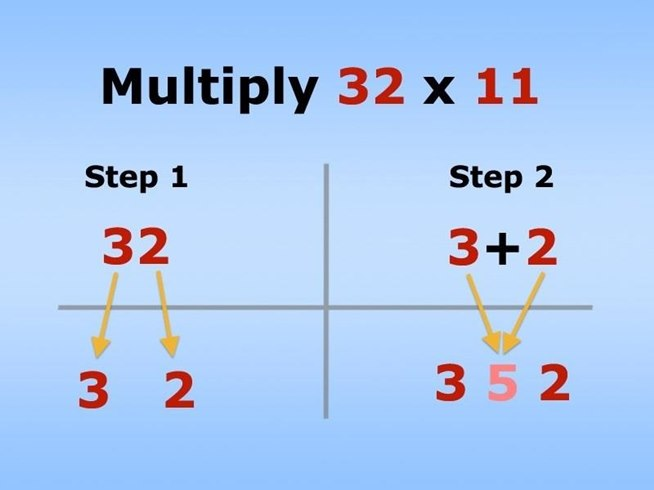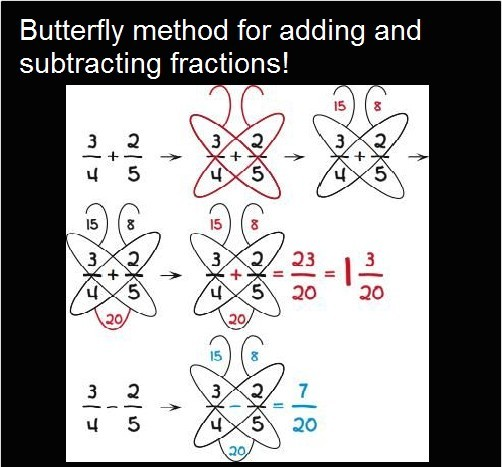# 12 Useful Math Hacks That They Didn’t Teach You In School

Whether we realize it or not, we solve math problems on a daily basis. Math is a difficult and tricky science that we all had to study at school. These handy math tricks can help you figure out each of these situations quickly — and without having to resort to pulling out the calculator. Going through these simple yet efficient math hacks would make you want to go back in time and improve your grades. Click below link for more math hacks.### 1. How To Convert Fahrenheit to CelsiusVia reddit/u/quaxonVia imgur / MoonnMan

### 3. How To Multiply By 11

Finally! I can now multiply more than single digits by 11 in my head.Via WonderHowTo

### 4.How To Figure Out Multiples Of NineCount up in the tens column and down in the ones column.Via Pinterest / Charles Stirton.

### 5.How to Add And Subtract FractionsVia imgur / ayounes

### 6.How to multiply numbers Use Lines.

How to use lines to multiply 13*23=299.12 Useful Math Hacks That They Didn’t Teach You In School